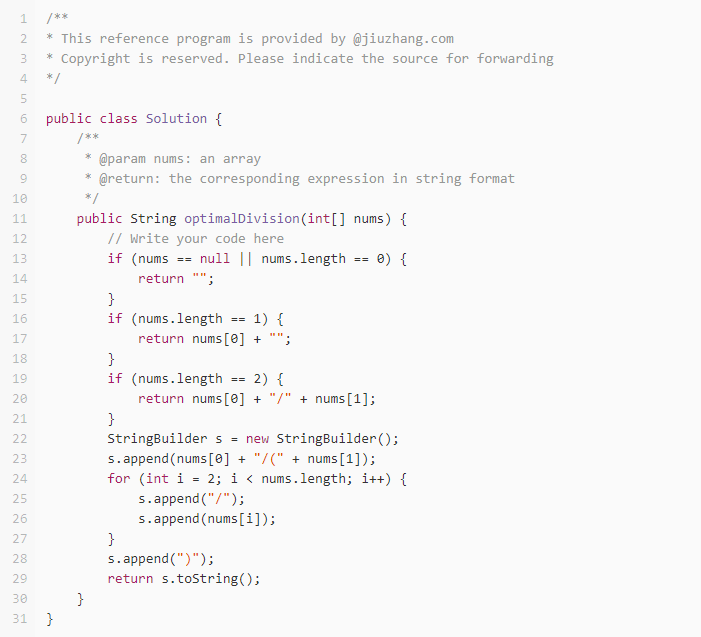# 【LintCode题解|最优除法】

【题目描述】

1.输入数组的长度范围为[1, 10].
2.数组元素范围为[2, 1000].
3.对于每个测试样例，只有一个最优解.

【题目样例 】

1000/(100/10/2) = 1000/((100/10)/2) = 200

1000/(100/10)/2 = 50
1000/(100/(10/2)) = 50
1000/100/10/2 = 0.5
1000/100/(10/2) = 2

【题目解析 （Java）】